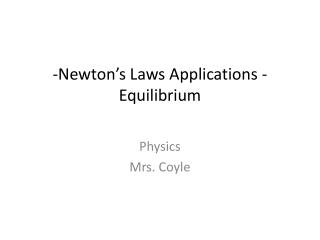DownloadDownload Presentation-Newton’s Laws Applications - Equilibrium

# -Newton’s Laws Applications - Equilibrium

Télécharger la présentation## -Newton’s Laws Applications - Equilibrium

- - - - - - - - - - - - - - - - - - - - - - - - - - - E N D - - - - - - - - - - - - - - - - - - - - - - - - - - -
##### Presentation Transcript

1. -Newton’s Laws Applications -Equilibrium Physics Mrs. Coyle

2. Outline • Solving Newton’s Laws Problems where the objects are accelerating (involving tension, normal force). • Static Equilibrium including the Suspended Sign, Inclined Plane , Atwood Machine.

3. M1 = 20kg Example 1 • Two boxes are on a horizontal table. They are connected by a string. Find the acceleration of each box and the tension on the string, if a force of 10N is applied horizontally. F=10N M2= 5kg

4. Equilibrium: a)Static, b)Dynamic ∑F = 0 a=0 • Static Equilibrium: the object is at rest, v=0. • Dynamic (Translational) Equilibrium: the object is moving at constant velocity, v=constant. • The Equilibrant is a force that is exerted on the system to bring it to equilibrium.

5. Suspended Sign ProblemHow do you calculate the tensions in the strings?-How do the angles affect the tensions?

6. Example 1 a)Calculate the tension in each string. The mass of the sign is 2kg and the angles formed between each string and the sign are 300. b)If the angles were 10o, what is the tension and how does it compare with the answer to a)? Answer: a) T1 = T2 = 20N b) T1 = T2 = 58N

7. In which case is the tension greater? http://wwwrcamnl.wr.usgs.gov/sws/SWTraining/cableways/design.htm

8. Do the angles affect the tension? • Examples: • Telephone or electrical wires. • Picture frame wire.

9. Example 2 T1 T2 A sign is suspended as shown above.   Calculate the tension in each string. Answer: T1 =289N, T2 =144N

10. ө Inclined Plane Normal, N Weight, mg

11. 30o Example 1- Find the acceleration. F=35 N m= 5kg 15° Frictionless plane.

12. Atwood Machine • Express the acceleration in terms of m, M and g. http://www.staff.amu.edu.pl/~romangoc/graphics/M3/7-atwood-machine/M3-7-fig1.gif

13. M1 Find the acceleration and the tension (assume frictionless surface). M2